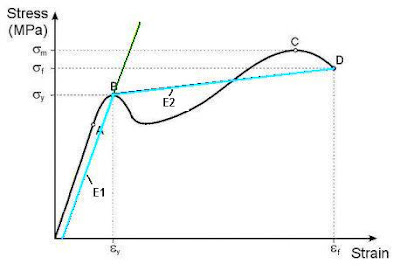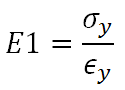## Monday, June 1, 2009

### Bi-Linear Material Models

I don't do FEA very often. Most of my designs are fairly simple with hearty factors of safety built in. Therefore, I can get away with closed-form solutions using standard Mechanics of Materials theory, including Roark's Formulas for the more complex boundary conditions, conservative estimates to simplify the problem, and a good use of professional judgement.

But, when I need to run FEA, it is because the problem is too complex for me to make accurate judgements or conservative simplifications. Thus, I need a fairly accurate representation. Most of all, I may be at the point where I pass yield. Running an FEA simulation using the standard linear material models is not sufficient. Yet, for a first-pass analysis I also don't need a fully defined model. I need a good approximation with a fast run-time.

Enter, the bi-linear material model.The figure above is of a typical stress-strain curve for steel.
The cyan colored line with the slope E1 is your standard Modulus of Elasticity in the elastic range as determined by the .2% offset to find the yield strength (sigma y) at the yield strain (epsilon y). The second cyan line with the slope E2 is the approximated elasticity in the plastic range of the material. It is approximated because, as you can obviously see, contains error above and below the line compared to the actual stress-strain curve. But, it is a much more accurate approximation as to the material's response to a load then continuing with the usual FEA analysis of linear materials as it would continue to follow the Modulus of Elasticity, projected as the green line.

Just like the Modulus if Elasticity (E1) is the slope of the line to the yield pointthe modulus of "plasticity" (E2) is the slope of the line from the yield point to the ultimate point.The benefit of using a bi-linear material model is
1. You can easily define the two equations of state with the data you already have from the material properties.
2. It solves relatively quickly because it uses the same matrix & solver as a linear static analysis, but changes the modulus value if the yield point is reached.
3. It is more accurate than assuming a non-yielding analysis.

The biggest problem with the bi-linear material model is that it is considered a nonlinear material model. For most FEA applications, that requires me to purchase the nonlinear package. In other words, to get a reasonable approximation, I have to spend a whole lot of money for material models I'll never use. It's not like I'm defining the equations of state for rubbers or amorphous solids. I'm just approximating the stress strain curve of isotropic materials beyond yield with a linear function. How is that nonlinear?

So why don't "express" versions of FEA software - the ones that come with the CAD software - have a the ability to solve bi-linear materials models without forcing me to purchase a bloated piece of non-linear material code I won't use? Bi-Linear material models are great for first-pass analysis and ballpark figures. Given enough margin for error, they can even be used for final approvals, but they are more in-line with comparative analysis and "warm fuzzies." Why not include them with express FEA solvers?